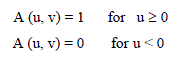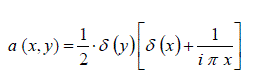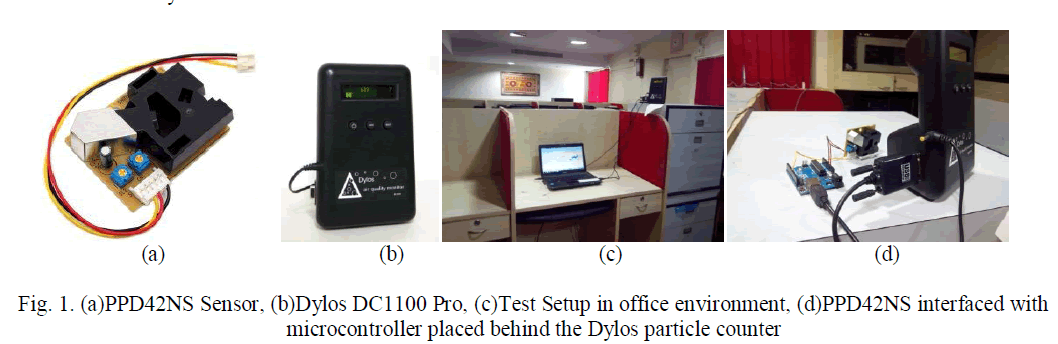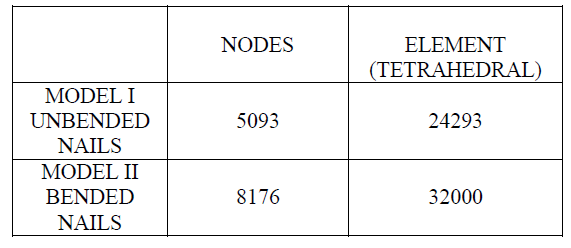ISSN ONLINE(2319-8753)PRINT(2347-6710)

All submissions of the EM system will be redirected to Online Manuscript Submission System. Authors are requested to submit articles directly to Online Manuscript Submission System of respective journal.

# Numerical Solution of the Navier-Stokes Equations at High Reynolds Numbers

 Blanca Bermúdez1, W. Fermín Guerrero S., 2 and A. Rangel-Huerta1 Benemérita Universidad Autónoma de Puebla, Facultad de Ciencias de la Computación, Puebla, México Benemérita Universidad Autónoma de Puebla, Facultad de Ciencias Físico- Matemáticas, Puebla, México Related article at Pubmed, Scholar Google

Visit for more related articles at International Journal of Innovative Research in Science, Engineering and Technology

## Abstract

In this work, we are working with the unsteady Navier-Stokes equations in the stream function-vorticity formulation. In order to show that thenumerical schemes used are able to handle highReynolds numbers, we are reporting results for the well known problem of the un-regularized driven cavity. We are dealing with Reynolds numbers in the range of 7500 ≤Re ≤ 50000. The results shown here are obtained using two numerical schemes, the first one, is based on a fixed point iterative process (see ) applied to the elliptic nonlinear system that results after time discretization. The second scheme (see , ) which, as we are going to show in the results, is faster than the first one, solves the transport type equation appearing in the Stream function-vorticity formulation of the Navier-Stokes equations using matrixes A and B; the first one resulting from the discretization of the Laplacian term appearing in the equation, and the second one resulting from the discretization of the advective term. Both schemes, for this problem, have been robust enough to handle such high Reynolds numbers, but the second one has proved to be much faster, especially for high Reynolds numbers. In  it has already been said that even though turbulence is a tri-dimensional phenomenon, two-dimensional flows at high Reynolds numbers give some clues of transition to real turbulence.

### Keywords

Navier-Stokes equations, Stream Function-vorticity formulation, high Reynolds numbers, fixed point iterative process.

### INTRODUCTION

In this paper we are presenting numerical results for Reynolds numbers in the range of 7500≤ Re ≤ 50000. Results for high Reynolds numbers have already been presented in the bibliography. Just to mention some of them: In  the range 10000 ≤Re≤ 20000 is presented; in , with primitive variables, the range 25000 ≤ Re ≤ 40000, and in  the range 400 ≤ Re ≤5000 to capture the steady state flow and the range10000 ≤ Re ≤ 20000 for time-dependent flows.
In the case of this work, we are using the stream function-vorticity formulation of the Navier-Stokes equations, and, as already mentioned in the abstract, results are obtained using two numerical schemes. The first one  is a simple numerical scheme for the unsteady Navier-Stokes equations in stream function and vorticity variables, based on a fixed point iterative process already used in the bibliography. This scheme worked very well, as shown in , , , , but the processing time was, in general, very large, especially for high Reynolds numbers. The second scheme discussed in , , works not only with the symmetric and positive matrix A resulting from the discretization of the Laplacian, but also with the matrix B resulting from the discretization of the advective term.
In the case of moderate Reynolds numbers, for instance Re ≤ 7500, the flow approaches to an asymptotic steady state as t tends to ∞, but in the case of high Reynolds numbers, such as the ones presented here, the solution seems to be time-dependent.
As the Reynolds numberincreases the mesh has to be refined and a smaller time step has to be used: numerically, by stability matters andphysically, to capture the fast dynamics of the flow.It hasalready been pointed out in  that to get the right vorticitycontours is more difficult than to get the rightstreamlines of the stream function, dueto oscillations appearing on the top right corner of the cavity because of insufficient mesh refining. So, in this work, we are going to present results for the vorticity contours.

### THE MATHEMATICAL MODEL OF THE PROBLEM### CONCLUSIONS

We are presenting two efficient numerical schemes for solving the Navier-Stokes in the Stream function-vorticity formulation. The idea of the fixed point iterative method was to work with a symmetric positive definite matrix (matrix A resulting from the discretization of the Laplacian term). This method showed to be robust enough to handle high Reynolds numbers, but computing time was, in general, very large. That is why we seek to reduce computing time, and implemented the second method.
Results with both methods, agree very well with those reported in the bibliography , , , but with the second method we were able to reduce computing time by almost three times, especially when increasing the Reynolds number. In this case, as we have said, smaller values of h have to be used, numerically for stability matters and physically, to capture the fast dynamics of the flow.
As can be seen from the results, fluid flows, at high Reynolds numbers approach two dimensional turbulence, caused by the recirculation in the driven cavity problem.The numerical results shows that the two dimensional flow, at high Reynolds numbers, presents great vorticity structure coming from recirculation of cavity. Under this circumstance, we still look forward to reduce the computing time of calculation. Results for even higher Reynolds numbers are also being obtained but these results will be reported in a future work.

### References

1. Nicolás, A., “A finite element approach to the Kuramoto-Sivashinski equation”, Advances in Numerical Methods, SIAM,1991.

2. Bermúdez, B., Juárez, L., “Numerical solution of an advection-diffusion equation”,InformaciónTecnológica, ,Vol. 5, No.1 , pp. 151–160, 2014.

3. Bermúdez, B., Posadas, R., “Another way of solving the Taylor Vortex and the Driven Cavity problem in the Stream function-vorticity formulation”, Proceedings WCCM XI, ECCM V, ECFD VI. pp 3746-3755, 2014.

4. Nicolás-Carrizosa, A., Bermúdez-Juárez, B., “Onset of two dimensional turbulence with high Reynolds numbers”, Proceedings of the IV International Conference on Computational Methods for Coupled Problems in science and engineering, pp. 1-5, 2011.

5. Bermúdez, B., Nicolás,A., Órtiz,C., “On Numerical solutions of the Navier-Stokes equations in Stream function-vorticity variables at high Reynolds numbers”, Proceedings of the Fifth World Congress on Computational Mechanics, Viena, Austria, 2001.

6. Nicolás, A., Bermúdez, B.,“ On some Numerical Solutions of the time dependent Navier-Stokes equations at High Reynolds numbers”, Proceedings of the Fifth World Congress on Computational Mechanics, Viena, Austria, 2001.

7. Nicolás, A., Bermúdez, B., “2D incompressible viscous Flows at moderate and high Reynolds numbers”, CMES, Vol. 6 No. 5, pp. 441-451, 2004.

8. Bermúdez, B., Nicolás, A., Sánchez F. J., Buendía E., “Operator Splitting and upwinding for the Navier-Stokes equations”, Computational Mechanics, Vol. 20, No. 5, pp. 474-477, 1997

9. Nicolás, A., Bermúdez, B., 2D Thermal/Isothermal incompressible viscous flows”, International Journal for Numerical Methods in Fluids, Vol. 48, pp. 349-366, 2005.

10. Nicolás, A., Bermúdez, B., “Isothermal/Thermal incompressible viscous Fluid Flows with the Velocity-Vorticity Formulation, InformaciónTecnológica, Vol. 21, No. 3., pp-39-49, 2010.

11. E. J. Dean, R.,Glowinski and O.,Pironneau, “ Iterative solution of the Stream function-Vorticity formulation of the Stokes problem, application to the Numerical simulation of incompressible viscous flows#, Computational Methods Appl. Mech. Engrg., pp. 117-155, 1991.

12. Goyon, O., High Reynolds number solutions of the Navier-Stokes equations using incremental unknowns”, computational Methods appl. Mech. Engrg., pp. 319-335, 1996.